# Gamedev Tutorial: Trigonometry Basics – Sine & Cosine

Source files and future updates are available on Patreon.

This post is part of my Gamedev Tutorials Series.

## Overview

Trigonometry is a very essential building block to a huge portion of game math. That’s why I’ve chosen this topic for the first tutorial of my new Gamedev Tutorials series. Having a solid understanding of basic of trigonometry can go a long way for game development. It is used extensively in game problem solving.

In this tutorial you’ll learn:

• A geometric interpretation of two basic trigonometric functions: sine & cosine.
• The comparison of two different angle units: degrees & radians.
• Some basic properties of sine & cosine.
• How to move and arrange things in a circular fashion:
• How to move things in a spiral fashion:
• How to create simple harmonic motion:
• How to create damped spring motion:
• How to create pendulum motion:
• How to generate hovering motion:

## Geometric Interpretation of Sine & Cosine

Let’s look at the unit circle, a circle with a radius of 1 centered at the origin.

Now pick a point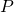on the circle. The line segment between this point and the origin forms an angle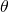(theta) between it and the X axis (positive X direction).

What’s shown here is actually a way to geometrically express the 2 basic trigonometric functions: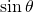(sine of theta) and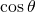(cosine of theta). The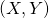coordinates of this pointare exactly.

So, to recap, the two trigonometry functionandare, respectively, the Y and X coordinates of a point on the unit circle, whereis the angle from the X axis (positive X direction) to the line segment between the point and the origin.

Sinceand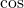are functions, the proper notation should include parenthesis around the input: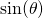and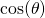, but many people and literature just ignore the parenthesis and write them asand.andare functions that take one single input (an angle) and output a single value between -1 and 1. If you think about it, this output range makes sense: the X and Y coordinates of a point on the of the unit circle can never go outside of the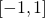range.

If the angleincreases at a constant rate, we can plot the value of the point’s X and Y coordinates individually over time.

If we compare the plots side-by-side in the form of angle-vs-value, we can see they are the same periodic curve in a wave-like shape, but offset by a fourth from each other.

The period of these functions is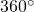, so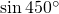gives the same value as. This makes sense, because rotating an extrapast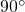would bring us back to the same angle.

The angle passed into the trigonometric functions can be in two different units: degrees and radians. Most people are familiar with degrees and its upper-little-circle notation. For instance, the right angle (90 degrees) is written as. Do beware thatis not the same as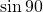. If the degree notation is not present, the angle’s unit is actually regarded as radians.is equivalent to(pi) radians, whereis the famous mathematical constant, “the ratio of a circle’s circumference to its diameter”, approximately equal to 3.14. Hence, an angle of 1 radian is approximately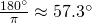, almost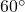. As a sanity check, entering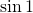on an engineering calculator (with angle units set to radians) will give us approximately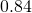, which is indeed close to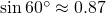.

Here are some common degree-to-radian mappings: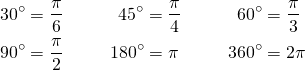In Unity, theandfunctions are called via Mathf.Sin and Mathf.Cos, respectively. Beware that these functions take input in radians, so if you want to compute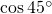, don’t write:

// this is actually cosine of 45 radians!
float cos45Deg = Mathf.Cos(45.0f);


45 radians is about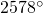. A full revolution ofis equivalent to no rotation at all.divided bygives us a remainder of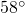, which is the equivalent angle ofand is different from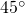.

To compute, write this instead:

// covert to radians
float cos45Deg = Mathf.Cos(45.0f * Mathf.PI / 180.0f);


Or use constants that help convert between degrees and radians.

float cos45Deg = Mathf.Cos(45.0f * Mathf.Deg2Rad);


In tools like the Unity editor, expressing angles in degrees is more user friendly, because most people can immediately picture what aangle looks like. However, in the context of math and programming, many people, myself included, prefer sticking with radians.

One useful thing about radians is that it trivializes calculating arc length from a given radius and angle. Let’s say we want to calculate the length of an arc of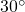, or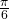radians, from a circle of radius 2.

If computed using degrees, first the whole circumference is calculated using the formula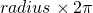, and then it’s multiplied by the ratio ofout of: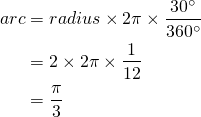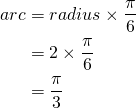The circle’s circumference formula agrees nicely with the arc length formula in radians. Since one full circle is basically an arc with an angle of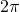radians, the length of such arc is, exactly the same as the circle’s circumference formula.

## Basic Properties of Sine & Cosine

Now let’s look at some basic properties of sine & cosine that can come in handy in future mathematical derivations.

Since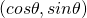are coordinates of a point on the unit circle, the point’s distance from the origin is always 1, regardless of the angle. The Pythagorean theorem states that the distance of the pointfrom the origin is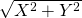. From there we can get this identity (equation that is always true):The squares ofandare written as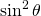and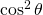, respectively. People write them that way probably because they are too lazy to write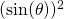and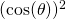.

Recall the side-by-side comparison of the sine and cosine plots.

You can see that the cosine curve basically is the sine curve shifted to the left by, or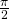radians. This means we can get these identities that convert between the two: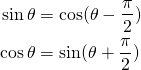## Moving in Circles & Spirals

Now that we’ve seen thatare 2D coordinates of a point on the unit circle, we can start playing with some basic circular motion in Unity.

The code below moves an object around a circle at a constant rate:

obj.transform.position =
new Vector3
(
0.0f
);


The code below moves 12 objects around a circle at a constant rate, and the objects are equally spaced out around the circle:

float baseAngle = Rate * Time.time + angleOffset;
for (int i = 0; i < 12; ++i)
{
float angleOffset = 2.0f * Mathf.PI * i / 12.0f;
aObj[i].transform.position =
new Vector3
(
0.0f
);
}


Combining circular motion with movement in the Z direction, we can create a spiral motion in 3D:

obj.transform.position =
new Vector3
(
ZSpeed * Time.time
);


## Simple Harmonic Motion (S.H.M.)

We’ve seen this plot of cosine versus angle:

What if we plug cosine into the X coordinate of an object?

float x = Mathf.Cos(Rate * Time.time);
obj.transform.position = Vector3(x, 0.0f, 0.0f);


This is what we get:

This kind of oscillating motion that matches a sine-shaped curve, a.k.a. sinusoid, is known as simple harmonic motion, or S.H.M.

Sincestarts at zero, the object’s X coordinate starts at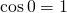. If we use, the X coordinate would start at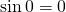.

The input angle passed in to the sine and cosine functions are called the phase. Typically, if the phase passed in is a constant multiple of time, many people write it as, where(omega) is called the angular frequency (in radians per second), andis the time. For example,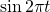would produce a simple harmonic motion that oscillates one full cycle every second.

What if we scale this motion by an exponentially decreasing factor?

float s = Mathf.Pow(0.5f, Decay * Time.time);
float x = Mathf.Cos(Rate * Time.time);
obj.transform.position = Vector3(s * x, 0.0f, 0.0f);


Now the object moves in a damped spring motion:

## Pendulum Motion

Instead of plugging a sinusoid into an object’s X coordinate, what if we plug it into the angle for the circular motion example above?

float baseAngle = 1.5f * Mathf.PI; // 270 degrees
float halfAngleRange = 0.25f * mathf.PI; // 45 degrees
float c = Mathf.Cos(Rate * Time.time);
float angle = halfAngleRange * c + baseAngle;
obj.transform.position =
new Vector3
(
0.0f
);


The object now moves in a pendulum motion:

We can treat this as the circular motion’s angle being in a simple harmonic motion.

## Hovering Motion

As a bonus example, here is UFO Bunny, a character from Boing Kit, my bouncy VFX extension for Unity.

We can apply staggered simple harmonic motion to her X, Y, and Z coordinates separately.

Vector3 hover =
new Vector3
(
RadiusX * Mathf.Sin(RateX * Time.time + OffsetX),
RadiusY * Mathf.Sin(RateY * Time.time + OffsetY),
RadiusZ * Mathf.Sin(RateZ * Time.time + OffsetZ)
);

obj.transform.position = basePosition + hover;


And this creates a hovering motion.

And the hover offset can be used to compute a tilt rotation. This is beyond the scope of this tutorial, so I’ll just leave the code and results here.

obj.transform.rotation =
baseRotation
* Quaternion.FromToRotation
(
Vector3.up,
-hover + 3.0f * Vector3.up
);


## Summary

That’s it!

We have seen howandcan be geometrically defined as coordinates of a point on the unit circle.

Also, we have seen the difference between the two angle units: degrees and radians.

Finally, we now know how to moves things in circles and spirals, as well as oscillating things in simple harmonic motion, damped spring motion, pendulum motion, and hove motion.

I hope this tutorial has helped you get a better understanding of the 2 basic trigonometric functions: sine & cosine.

In the next tutorial, I will introduce one additional basic trigonometric function: tangent, as well as talk about more applications of all these 3 functions.

Until then!

If you’ve enjoyed this tutorial and would like to see more, please consider supporting me on Patreon. By doing so, you can also get updates on future tutorials. Thanks!Physics / Graphics / Procedural Animation / Visuals
This entry was posted in Gamedev, Math, Programming and tagged , , , , , . Bookmark the permalink.

### 8 Responses to Gamedev Tutorial: Trigonometry Basics – Sine & Cosine

1.Christopher Sisk says:

I’ve spent the better part of 20 years designing and programming games, and in all this time this is the first time I’ve properly ‘grokked’ sin, cosine, and radians. Incredibly concise, to the point, and easy to understand with practical examples demonstrating the actual function and use of the methods.

One small comment, for whatever reason I was confused for a while by “You can see that the cosine curve basically is the sine curve shifted to the left by 90^\circ, or \frac{\pi}{2}.”. It might be slightly more clear for dotards such as myself if you explicitly state that this is in radians, even if you did earlier state that omitting degrees implies this.

•Allen Chou says:

Good point. I’ve added the explicit units. Thanks.

2.Sammy says:

I’m going to provide some blunt feedback on this, and I hope it is taken in the spirit it is intended, which is to help you improve. Background: I’m always looking for simple explanations of advanced ideas to show my primary school aged children – I want to introduce my kids to those ideas early but without the stress and hassle of doing worked problems.

This is an excellent summary but a terrible tutorial. It’s such an excellent summary that I’ve bookmarked it. It’s such a terrible introduction/tutorial that there is no way I’m exposing my kids to it until they have a solid grasp of those 2 functions. If you haven’t seen this stuff before this tutorial would be incredibly confusing. If you have, you realize what an elegant and conscise summary and demo you’ve constructed.

•Allen Chou says:

Thanks for the feedback! I will keep this in mind when writing future tutorials.

3.Dmytro Kiro says:

Awesome! Keep it going!

4.Some Guy says:

Finally I was able to learn how to use SIN and COS.

5.Ishaan Sutaria says:

Great tutorial!

6.apelmon says:

Awesome article! Thanks a lot.

This site uses Akismet to reduce spam. Learn how your comment data is processed.